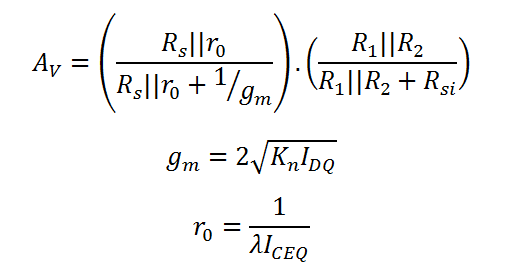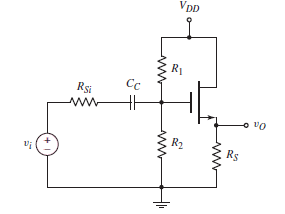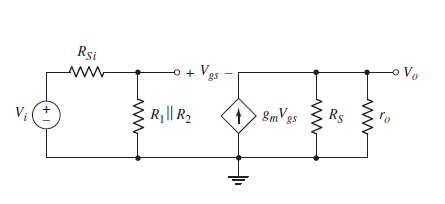# Small Signal Analysis of Common Drain (CD) MOSFET Amplifier

This CalcTown calculator calculates the small signal voltage gain of a Common Drain (CD) MOSFET amplifier, working in the saturation mode. A Common Drain MOSFET Circuit is also called a Source Follower circuit because any change in the Drain voltage is reflected in the Source Voltage.

Its small output resistance makes it desirable when the circuit is to act as an ideal voltage source and drive a load circuit

* Please note that body effect has been taken into consideration only for calculating the small signal output resistance and not for the current calculation in DC analysis.

K-Ohm
K-Ohm
K-Ohm
K-Ohm
uA/V2
V-1

#### Result

K-Ohm
uA/V
V/V